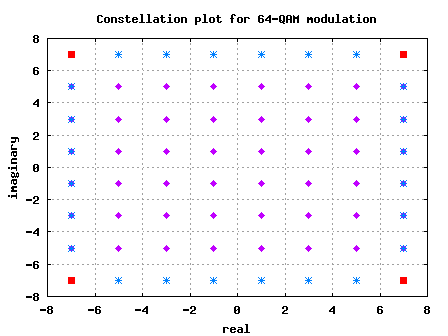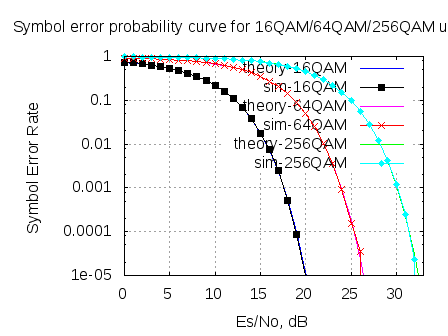- DSP log - http://www.dsplog.com -

Symbol Error rate for QAM (16, 64, 256,.., M-QAM)

Posted By Krishna Sankar On January 1, 2012 @ 4:34 pm In Modulation | 44 Comments

In May 2008, we derived the theoretical symbol error rate for a general M-QAM modulation (in  Embedded.com DSPDesignLine.com  and dsplog.com ) under Additive White Gaussian Noise. While re-reading that post, felt that the article is nice and warrants a re-run, using OFDM as the underlying physical layer. This post discuss the derivation of symbol error rate for a general M-QAM modulation. The companion Matlab script compares the theoretical and the simulated symbol error rate for 16QAM, 64QAM and 256QAM over OFDM in AWGN channel.

Enjoy and HAPPY NEW YEAR 2012 !!!

Quadrature Amplitude Modulation (QAM) schemes like 16-QAM, 64-QAM are used in typical wireless digital communications specifications like IEEE802.11a IEEE802.16d . In this post let us derive the equation for probability of symbol being in error for a general M-QAM constellation, given that the signal (symbol) to noise ratio is  .

## The general M-QAM constellation

The number of points in the constellation is defined as,  where  is the number of bits in each constellation symbol. In this analysis, it is desirable to restrict  to be an even number for the following reasons (Refer Sec 5.2.2 in [DIG-COMM-BARRY-LEE-MESSERSCHMITT]):

1. Half the bits are represented on the real axis and half the bits are represented on imaginary axis. The in-phase and quadrature signals are independent  level Pulse Amplitude Modulation (PAM) signals. This simplifies the design of mapper.

2. For decoding, symbol decisions may be applied independently on the real and imaginary axis, simplifying the receiver implementation.

Note that the above square constellation is not the most optimal scheme for a given signal to noise ratio.

## Average energy of an M-QAM constellation

In a general M-QAM constellation where  and  the number of bits in each constellation is even, the alphabets used are:

, where .

For example, considering a 64-QAM () constellation,

and the alphabets are

.

For computing the average energy of the M-QAM constellation, let us proceed as follows:

(a) Find the sum of energy of the individual alphabets

(b) Each alphabet is used  times in the M-QAM constellation.

(c) So, to find the average energy from  constellation symbols, divide the product of (a) and (b) by.The average energy is,

.

Plugging in the number for 64-QAM,
.

Plugging in the number for 16-QAM,
.

From the above explanations, it is reasonably intuitive to guess that the scaling factor of  which is seen along with 16-QAM, 64-QAM constellations respectively is for normalizing the average transmit power to unity.

## Finding the symbol error rate

For computing the symbol error rate for an M-QAM modulation, let us consider the 64-QAM constellation as shown in the figure below and extend it to the M-QAM case.Figure: Constellation plot for 64-QAM modulation (without the scaling factor of )

As can be seen from the above figure, there are three types of constellation points in a general M-QAM constellation:

(a) Constellation points in the corner (red-square)
The number of constellation points in the corner in any M-QAM constellation is always 4, i.e

(b) Constellation points in the inside (magneta-diamond)
The number of constellation points in the inside is,

.
For example with M=64, there are 36 constellation points in the inside.

(c) Constellation points neither at the corner, nor at the center (blue-star) The number of constellation points of this category is,
.

For example with M=64, there are 24 constellation points in the inside.

## Additive White Gaussian Noise (AWGN) channel

, where

is the energy,
is the normalizing factor,

is the transmit symbol and
is the noise.

Assume that the additive noise  follows the Gaussian probability distribution function,

with

mean  and

variance .

Symbol in the inside  (magenta-diamond)

The conditional probability distribution function (PDF) of given that the transmitted symbol is  :

.

As can be seen from the above figure, the symbol in the inside is decoded correctly only if real part of   lies inbetween 0 to 2 and the imaginary part of   lies inbetween 0 to 2. The probability of correct demodulation is,

.

The probability of the real component falling with in 0 to 2 can be found by integrating the probability distribution function of two parts:

(a) Find the probability that the real component lies from  to .

(b) Find the probability that the real component lies from  to .

(c) Given that the total probability is always 1, for finding the probability of the real component lies within  to , subtract the sum of (a) and (b) from 1.

Note: The complementary error function, .

Similarly,

.

From the above equations,

.

The probability of the symbol decoded being in error is,

.

Symbol in the corner  (red-square)

The conditional probability distribution function (PDF) of given that the transmitted symbol is  :

.

As can be seen from the above figure, the symbol in the inside is decoded correctly only if real part of   lies from  to  and the imaginary part of   lies from  to .

.

For finding the probability that the real component lies from  to , one can integrate the probability distribution function of the received symbol.

Similarly,

.

So, probability that the decoded symbol is correct given is transmitted is,

.

Now, the probability of the symbol decoded being in error is,

.

Symbol neither at the corner nor inside  (blue-star)

As can be seen from the above figure, the symbol in the inside is decoded correctly only if real part of   lies from  to  and the imaginary part of   lies from 0 to 2.

.

For finding the probability that the real component lies from  to , one can integrate the probability distribution function of the received symbol.

.

As described for the symbol in the inside scenario, the probability of the imaginary component falling with in 0 to 2 can be found by integrating the probability distribution function of two parts:

(a) Find the probability that the imaginary component lies from  to .

(b) Find the probability that the imaginary component lies from  to .

(c) As the total probability is 1, for finding the probability of the imaginary component lies within  to , subtract the sum of (a) and (b) from 1.

So the probability that the symbol is decoded correctly is,

The probability of error is,

.

Total symbol error probability

Given that we have computed the individual symbol error probability for each of the three types of constellation points, to find the joint symbol error rate we compute the average error i.e.

Pluging in the equations,
.

## OFDM modulation

Let us use the OFDM system loosely based on IEEE 802.11a specifications.

 Parameter Value FFT size. nFFT 64 Number of used subcarriers. nDSC 52 FFT Sampling frequency 20MHz Subcarrier spacing 312.5kHz Used subcarrier index {-26 to -1, +1 to +26} Cylcic prefix duration, Tcp 0.8us Data symbol duration, Td 3.2us Total Symbol duration, Ts 4us

## Simulation model

Simple Matlab/Octave script for computing the symbol error rate for  16QAM/64QAM and 256QAM modulation scheme in additive white gaussian noise channel over OFDM.## References

[DIG-COMM-BARRY-LEE-MESSERSCHMITT]Digital Communication: Third Edition, by John R. Barry, Edward A. Lee, David G. Messerschmitt 

URL to article: http://www.dsplog.com/2012/01/01/symbol-error-rate-16qam-64qam-256qam/

URLs in this post:

 Embedded.com: http://www.embedded.com/columns/technicalinsights/207801597?_requestid=88879

 DSPDesignLine.com: http://www.dspdeisgnline.com/

 dsplog.com: http://www.dsplog.com/2008/05/24/article-in-dspdesignlinecom-m-qam-symbol-error/